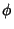Whewell Equation

An Intrinsic Equation which expresses a curve in terms of its Arc Lengthand Tangential Angle.

See also Arc Length, Cesàro Equation, Intrinsic Equation, Natural Equation, Tangential Angle

References

Yates, R. C. Intrinsic Equations.'' A Handbook on Curves and Their Properties. Ann Arbor, MI: J. W. Edwards, pp. 123-126, 1952.